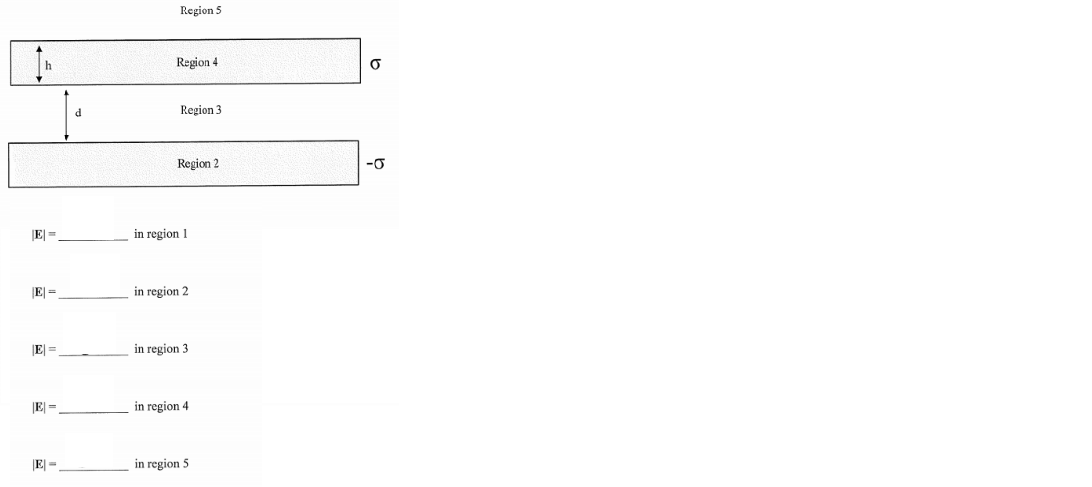# Problem: The two infinite, conducting plates of thickness = h and separation = d are oppositely charged. The charge per unit area on the top plate is σ , and the charge on the bottom plate is σ , where σ is a positive number. What is the magnitude of the electric field in each region depicted in the picture?

###### FREE Expert Solution
82% (395 ratings)
###### Problem Details

The two infinite, conducting plates of thickness = h and separation = d are oppositely charged. The charge per unit area on the top plate is σ , and the charge on the bottom plate is σ , where σ is a positive number. What is the magnitude of the electric field in each region depicted in the picture?